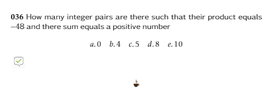# -gre.al.1 GRE Exam Integers Product of two integers is -48 but their sum is a positive number

• MHB
Gold Member
MHB
ok I don't don't know de jure on this so ...

is it just plug and play??

find factors of -48
$-1(48)=-48$
$-2(24)=-48$
$-3(16)=-48$
$-4(12)=-48$
$-6(8)=-48$
check sums for positive number
$-1+48=47$
$-2+24=22$
$-3+16=13$
$-4+12=8$
$-6+8=2$

it looks like c. 5

#### Attachments

•036.png
3.3 KB · Views: 62

Monoxdifly
MHB
is it just plug and play??

Well, if just plug and play can get you there faster, why bother with theoretical method?

Gold Member
MHB
mahalo;)

Last edited: